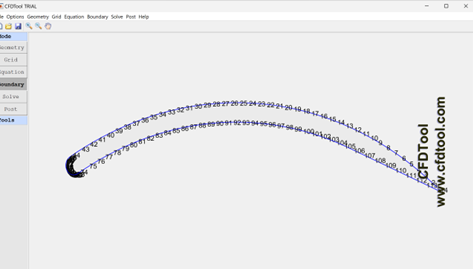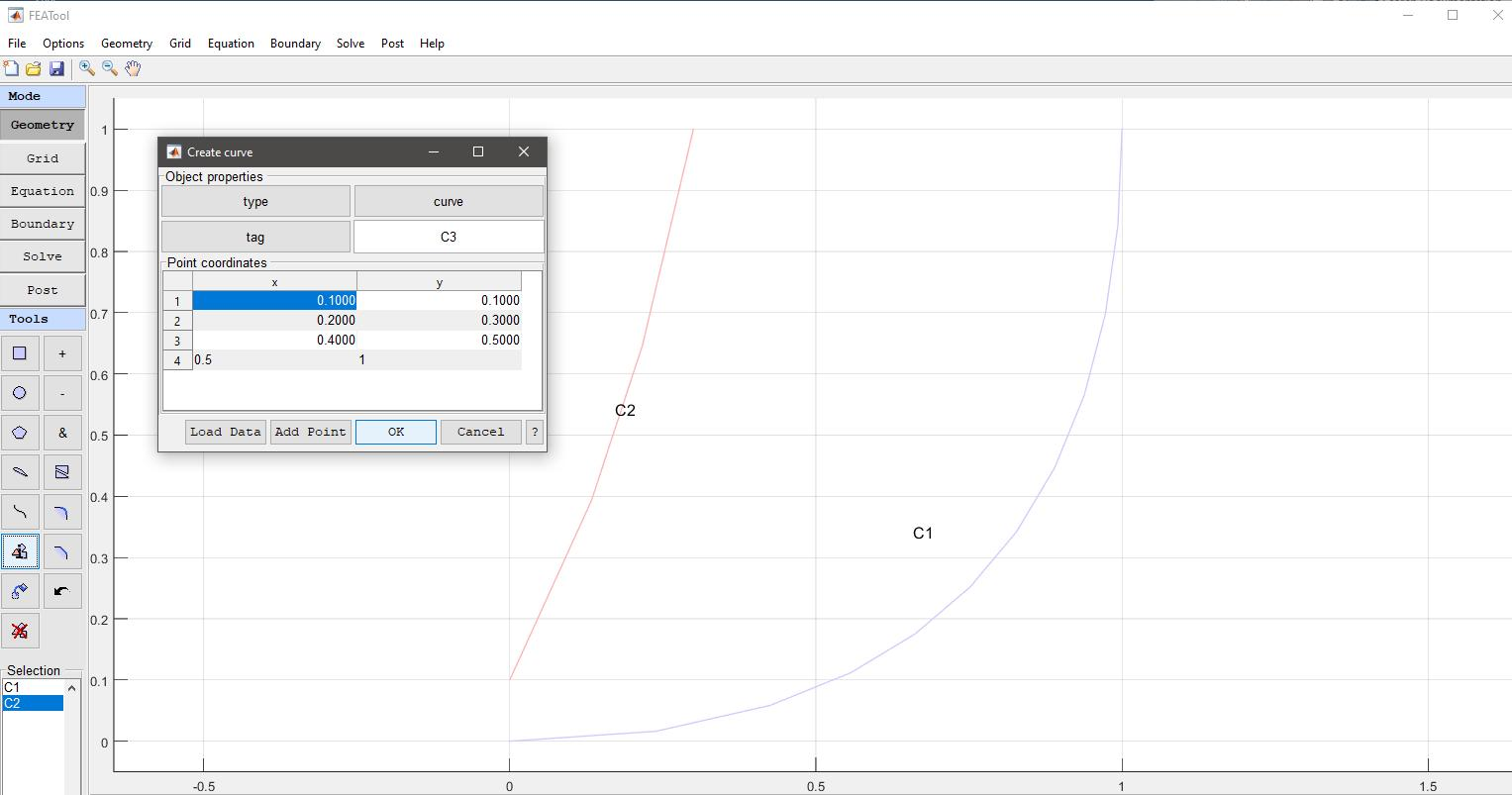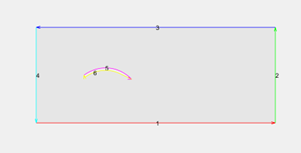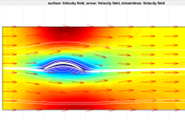# Modeling geometry using X Y maytrix from MatlabClassicListThreaded5 messagesOpen this post in threaded view
|

## Modeling geometry using X Y maytrix from Matlab

 Working with Matlab environment to generate cambered plate profile, I was looking forward importing these X Y variable to generate the 2D shape, and ultimately run cfd simulation. I was able to import the spline using a csv file where the date are stored, but cannot close the contour line and generate a 2D shape. The  NACA profile menu generates the shape via a dedicated program. Any suggestion to address the case. Thanks in advance.
Open this post in threaded view
|

## Re: Modeling geometry using X Y maytrix from Matlab

 Administrator Could you attach some example data from which you want to generate a shape which results in issues?
Open this post in threaded view
|

## Re: Modeling geometry using X Y maytrix from Matlab

 Thanks for reply. Being new to CFDtool, I did not find the solution straight away. With my csv file, as attached, using Polygon load data, I was able to create the complete foil shape.The main difference with the NACA profile program is the boundary format. In my case multiple segments, with the NACA generation profile 2 segments 1 for intrados, 1 for extrados) which makes it easier to compute function like the pressure coeffcient. Thanks
 Administrator It technically should be able to create a single spline/bezier curve from a series of points. If you upload the CSV data I can have a look.Thanks for the hints on loading data from "csv" file with spline function. I was able to generate the profile and test run using Navier Stokes equation, with turbulent boundary layer (Re=1E6). I have attached some images.Going forward, I would want to check drag and lift coefficient with respect to available data from airfoil database. I used the tutorial on the flow around the cylinder, with the calculation of the lift coefficient. Equation for the local drag force is set to : nx*p+miu*(-2*nx*ux-ny*(uy+vx)) Is there any reference documentation, apart from the tutorial, to explain the construction of this parametric equation from the integral equation ? I set the equation for the local lift force to : ny*p+miu*(-2*ny*uy-nx*(ux+vy)) but want to check the correctness. The combination with Matlab capacities makes CFD / FETools very powerfull. Thanks for the support